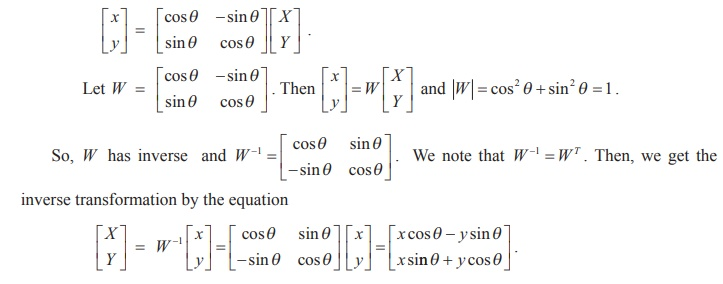Home | | Maths 12th Std | Application of matrices to Geometry

# Application of matrices to Geometry

There is a special type of non-singular matrices which are widely used in applications of matrices to geometry. For simplicity, we consider two-dimensional analytical geometry.

Application of matrices to Geometry

There is a special type of non-singular matrices which are widely used in applications of matrices to geometry. For simplicity, we consider two-dimensional analytical geometry.

Let  O  be the origin, and  x 'O x  and  y 'Oy be the  x -axis and y -axis. Let P be a point in the plane whose coordinates are (x, y) with respect to the coordinate system. Suppose that we rotate the x -axis and   y -axis about the origin, through an angle ╬Ė as shown in the figure. Let X 'OX and Y 'OY be the new X -axis and new Y -axis. Let  ( X ,Y )  be the new set of  coordinates of P with respect to the new coordinate system.  Referring  to  Fig.1.1, we get

x = OL = ON ŌłÆ LN = X cos ╬Ė ŌĆō QT =  X cos ╬Ė ŌłÆ Y sin ╬Ė ,

y = PL = PT + TL = QN + PT =  X sin ╬Ė + Y cos ╬Ė .These equations provide transformation of one coordinate system into another coordinate system.

The above two equations can be written in the matrix formHence, we get the transformation X = x cos╬Ė - y sin╬Ė , Y = x sin╬Ė + y cos╬Ė .

This transformation is used in Computer Graphics and determined by the matrixWe note that the matrix W satisfies a special property W -1 = WT ; that is, WW T = WTW = I .

### Definition 1.3

A square matrix A is called orthogonal if AAT = ATA = I.

### Note

A is orthogonal if and only if A is non-singular and AŌłÆ1 = AT .

### Example 1.11

Prove thatis orthogonal.

### SolutionSimilarly, we get ATA = I2 . Hence AAT = ATA = I2 ŌćÆ A is orthogonal.

### Example 1.12

If A =, is orthogonal, find a, b and c , and hence AŌłÆ1.

### Solution

If A is orthogonal, then AAT = AT A = I3 . So, we haveTags : Definition, Theorem, Formulas, Solved Example Problems | Inverse of a Non-Singular Square Matrix , 12th Mathematics : UNIT 1 : Applications of Matrices and Determinants
Study Material, Lecturing Notes, Assignment, Reference, Wiki description explanation, brief detail
12th Mathematics : UNIT 1 : Applications of Matrices and Determinants : Application of matrices to Geometry | Definition, Theorem, Formulas, Solved Example Problems | Inverse of a Non-Singular Square Matrix